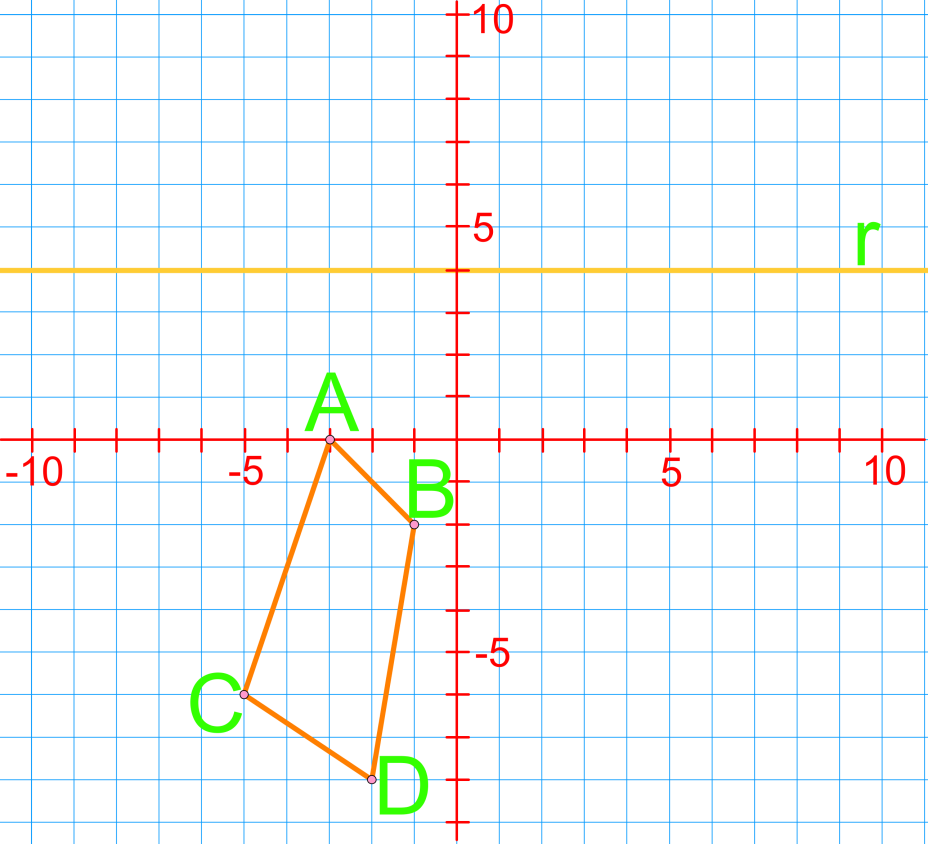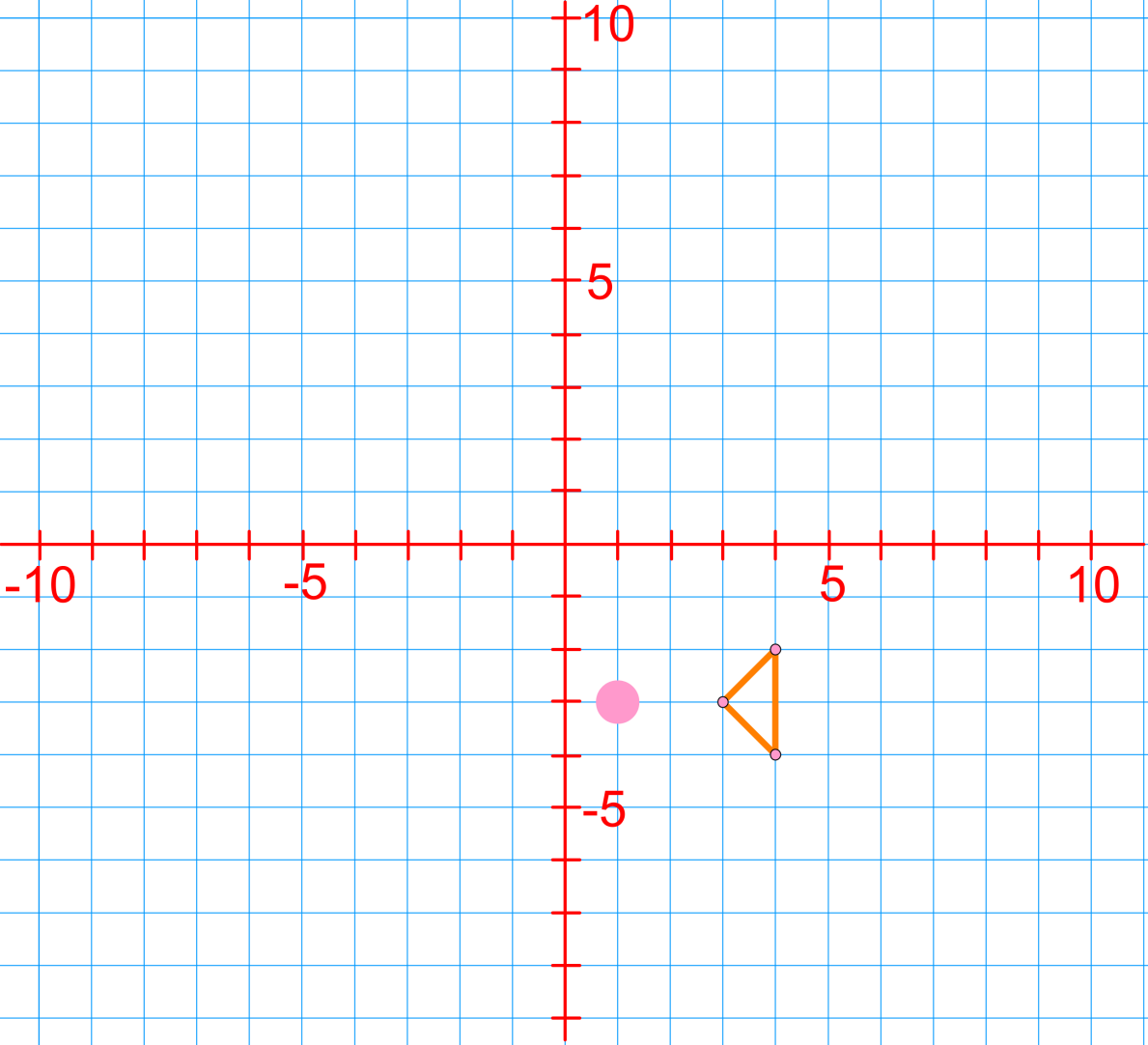# Introduction to transformations

### Introduction to transformations

When an object is reflected in a mirror, the line of reflection is the mirror. Reflections are a type of transformation. In this section, we will use our knowledge from sections on Cartesian plane and drawing on coordinate planes to show transformations of figures on coordinate planes. Along with reflections, translations and rotations are types of transformations. When showing translations, we always describe horizontal (left or right) movements before vertical (up or down) movements.

#### Lessons

• Introduction
Types of transformations:
What are translations, reflections and rotations?

• 1.
Draw the reflection image of the following diagram. The line of reflection is labeled $r$.• 2.
The diagram shows a quadrilateral. Rotate the figure 180 degrees counter clockwise about its centre of rotation, P.